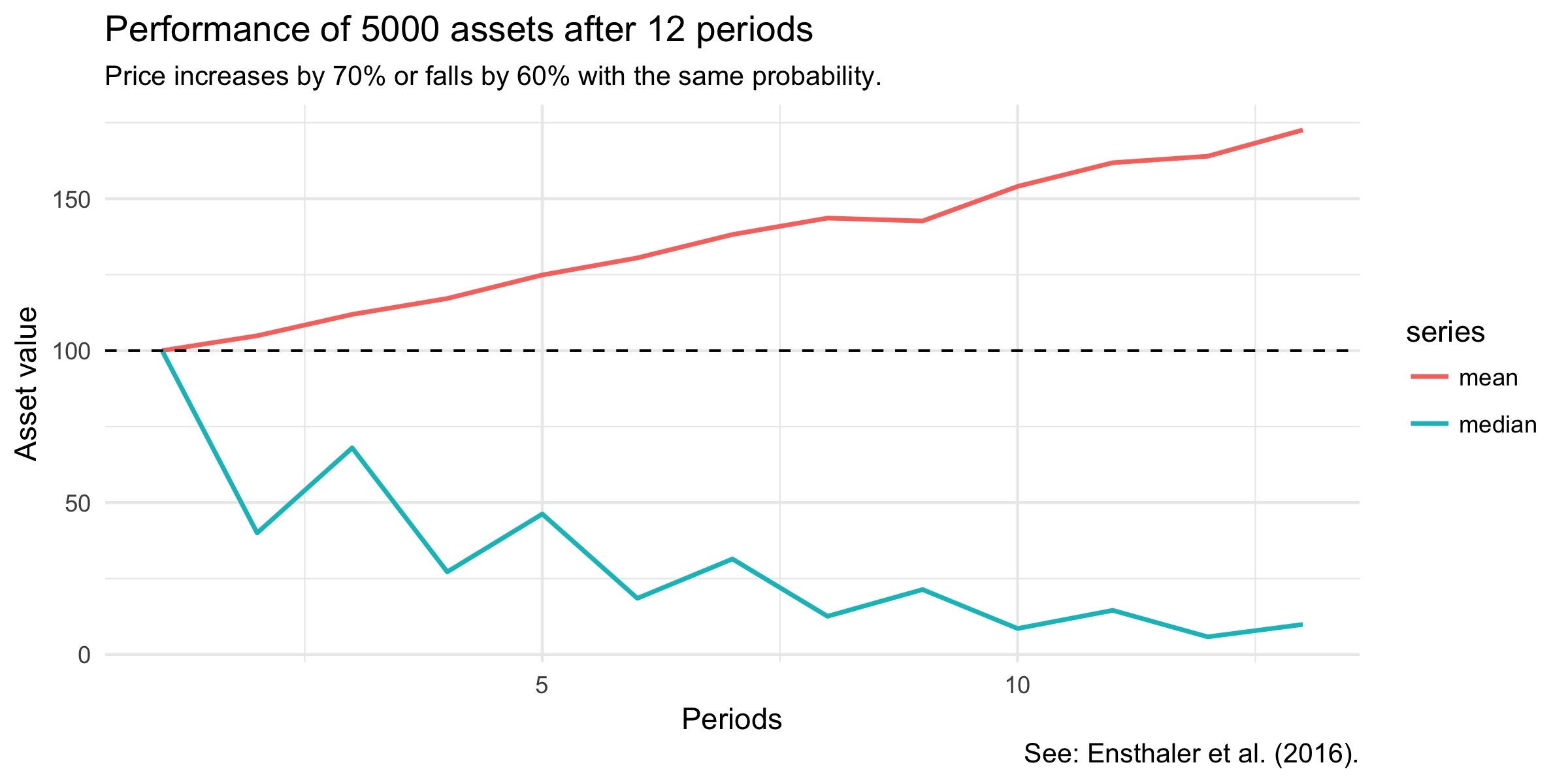# "Hidden Skewness"

In a recent paper (pdf), Ludwig Ensthaler, Olga Nottmeyer, Georg Weizsäcker and Christian Zankiewicz ask the following question:

• Consider an asset that’s worth 10,000 euros. For 12 periods the value of either rises by 70% or falls by 60% with equal probability. Now consider these five buckets: 1) below 6,400, 2) 6,400-12,800, 3) 12,800-19,200, 4) 19,200-25,600 or 5) above 25,600.
• The asset will be simulated for you and if you have guessed right and the asset ended up in your bucket, you get 20 euros. Else, you get nothing. Which bucket would you bet on?

My intuition would have been to go for the third bucket. But the right answer is the first bucket. The reason is that this question asks you what the most likely price for such an asset is, not for the average price. Ensthaler et al. offer the clue that one increase can’t compensate for one fall (0.4 · 1.7 = 0.68 < 1).

Let’s simulate this in R for many assets to see what this process is up to:

Next we extract some tidy statistics about our assets:

Now we can plot how prices develop over the 12 periods:

Which produces:All the assets start out at 100. The mean price of assets rises, but the median falls fast.

The authors conclude that people are bad at calculating compound interest rates and that we tend to neglect skewness.

Ensthaler, L., O. Nottmeyer, C. Zankiewicz and G. Weizsäcker (2016). “Hidden Skewness: On the Difficulty of Multiplicative Compounding under Random Shocks”. Management Science. doi